# Precalculus : Determine the Number of Positive and Negative Real Zeros of a Polynomial Using Descartes' Rule of Signs

## Example Questions

### Example Question #1 : Determine The Number Of Positive And Negative Real Zeros Of A Polynomial Using Descartes' Rule Of Signs

Determine the possible number of Positive and Negative Real Zeros of the polynomial using Descartes' Rule of Signs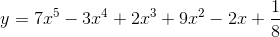Positive zeroes: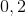Negative zeroes: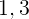Positive zeroes:Negative zeroes:Positive zeroes: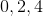Negative zeroes:Positive zeroes:Negative zeroes:Positive zeroes:Negative zeroes:Explanation:

In order to determine the positive number of real zeroes, we must count the number of sign changes in the coefficients of the terms of the polynomial. The number of real zeroes can then be any positive difference of that number and a positive multiple of two.

For the functionthere are four sign changes. As such, the number of positive real zeroes can beIn order to determine the positive number of real zeroes, we must count the number of sign changes in the coefficients of the terms of the polynomial after substituting for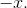The number of real zeroes can then be any positive difference of that number and a positive multiple of two.

After substituting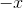we get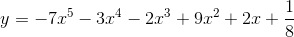and there is one sign change.

As such, there can only be one negative root.

### All Precalculus Resources